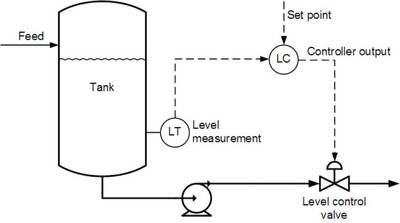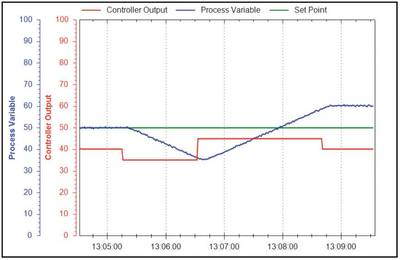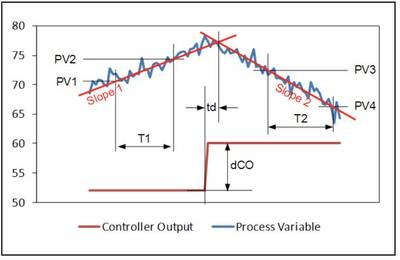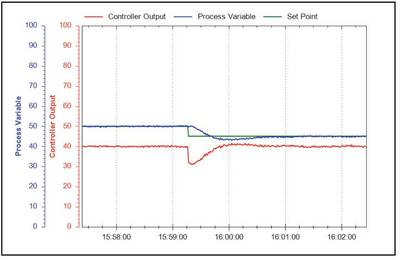# Industry News Display

2014-10-30   Control Engineering

## One-in-four control loops are regulating level, but techniques for tuning PID controllers in these integrating processes are not widely understood.

By: Lee PayneSince the first two PID controller tuning methods were published in 1942 by J. G. Ziegler and N. B. Nichols, more than 100 additional tuning rules have been developed for self-regulating control loops (e.g., flow, temperature, pressure). In contrast, fewer than 10 tuning methods have been developed for integrating (e.g., level) process types, though roughly one-in-four industrial PID loops controls liquid level.

The original Ziegler-Nichols tuning methods aimed for a super-fast response capability, which was achieved at the expense of control loop stability. However, a slight modification of these tuning rules improves loop stability while still maintaining a fast response to setpoint changes and disturbances. As most process experts will agree, stability is generally more important than speed.

Applicable process types

This modified Ziegler-Nichols tuning method is intended for use with integrating processes, and level control loops (Figure 1) are the most common example.Unlike a self-regulating process, an integrating process will stabilize at only one controller output, which has to be at the point of equilibrium. If the controller output is set to a different value, the process will increase or decrease indefinitely at a steady slope (Figure 2).

Note: This tuning method provides a fast response to disturbances in level and is therefore not suitable for tuning surge tank level control loops.

The modified Ziegler-Nichols tuning rules presented here are designed for use on a non-interactive controller algorithm with its integral time set in minutes. Dataforth's MAQ20 industrial data acquisition and control system uses this approach as do other controllers from a variety of manufacturers.

Procedure

To apply these tuning rules to an integrating process, follow these steps. The process variable and controller output must be time-trended so that measurements can be taken from them, as illustrated in Figure 3.

Step 1. Do a step test

a) Make sure, as far as possible, that the uncontrolled flow in and out of the vessel is as constant as possible.

b) Put the controller in manual control mode.

c) Wait for a steady slope in the level. If the level is very volatile, wait long enough to be able to confidently draw a straight line though the general slope of the level.

d) Make a step change in the controller output. Try to make the step change 5% to 10% in size, if the process can tolerate it.

e) Wait for the level to change its slope into a new direction. If the level is volatile, wait long enough to be able to confidently draw a straight line though the general slope of the level.

f) Restore the level to an acceptable operating point and place the controller back into automatic control mode.

Step 2. Determine process characteristicsBased on the example shown in Figure 3:

a) Draw a line (Slope 1) through the initial slope, and extend it to the right as shown in Figure 3.

b) Draw a line (Slope 2) through the final slope, and extend it to the left to intersect Slope 1.

c) Measure the time between the beginning of the change in controller output and the intersection between Slope 1 and Slope 2. This is the process dead time (td), the first parameter required for tuning the controller.

d) If td was measured in seconds, divide it by 60 to convert it to minutes. As mentioned earlier, the calculations here are based on the integral time in minutes, so all time measurements should be in minutes.

e) Pick any two points (PV1 and PV2) on Slope 1, located conveniently far from each other to make accurate measurements.

f) Pick any two points (PV3 and PV4) on Slope 2, located conveniently far from each other to make accurate measurements.

g) Calculate the difference in the two slopes (DS) as follows:

DS = (PV4 - PV3) / T2 - (PV2 - PV1) / T1

Note: If T1 and T2 measurements were made in seconds, divide them by 60 to convert them to minutes.

h) If the PV is not ranged 0%-100%, convert DS to a percentage of the range as follows:

DS% = 100 × DS / (PV range max - PV range min)

i) Calculate the process integration rate (ri), which is the second parameter needed for tuning the controller:

ri = DS [in %] / dCO [in %]

Step 3. Repeat

Perform steps 1 and 2 at least three more times to obtain good average values for the process characteristics td and ri.

Step 4. Calculate tuning constants

Using the equations below, calculate your tuning constants. Both PI and PID calculations are provided since some users will select the former based on the slow-moving nature of many level applications.

For PI Control

Controller Gain, Kc =  0.45 / (ri × td)

Integral Time, Ti =     6.67 × td

Derivative Time, Td = 0

For PID Control

Controller Gain, Kc = 0.75 / (ri × td)

Integral Time, Ti =     5 × td

Derivative Time, Td = 0.4 × td

Note that these tuning equations look different from the commonly published Ziegler-Nichols equations. The first reason is that Kc has been reduced and Ti increased by a factor of two, to make the loop more stable and less oscillatory. The second reason is that the Ziegler-Nichols equations for PID control target an interactive controller algorithm, while this approach is designed for a non-interactive algorithm such as is used in the Dataforth MAQ20 and others. (If you are using a different controller, make sure you find out which approach it uses.) The PID equations above have been adjusted to compensate for the difference.

Step 5. Enter the valuesKey your calculated values into the controller, making sure the algorithm is set to non-interactive, and put the controller back into automatic mode.

Step 6. Test and tune your work

Change the setpoint to test the new values and see how it responds. It might still need some additional fine-tuning to look like Figure 4. For integrating processes, Kc and Ti need to be adjusted simultaneously and in opposite directions. For example, to slow down the control loop, use Kc / 2 and Ti × 2.

With just a few modifications to the original Ziegler-Nichols tuning approach, these rules can be used to tune level control loops for both stability and fast response to setpoint changes and disturbances.

Lee Payne is CEO of Dataforth.

Additional reading: J.G. Ziegler and N.B. Nichols, Optimum settings for automatic controllers, Transactions of the ASME, 64, pp. 759-768, 1942.

ONLINE